Solubility Products of Slighly Soluble Metal Hydroxides Chemistry Lab Report

The Solubility Products of Slightly Soluble Metal Hydroxides

# Purpose

This experiment has two parts. The purpose of the first part of the experiment is to measure the solubility product constant, Ksp, for four slightly soluble hydroxides in water. The purpose of the second part of the experiment is to predict the pH of solutions of the hydroxides in-the presence of a common ion and to verifr your prediction by mixing the solutions and measuring their pH.

# Introduction

Solubility products are the equilibrium constants for the aqueous dissolution of slightly soluble ionic solids into their component ions. Metal hydroxides dissociate into metal ions and hydroxide ions: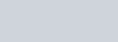Mn+(aq) + nOff(aq)

Metal hydroxides, with the exception of alkali metals and select alkaline earths, are only slightly soluble in water. In this experiment, you will explore the solubility of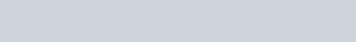Mg(OH)2, and Ba(OH)2. In the first part of the experiment, you will be provided with a saturated solution of each compound. Using a pH probe, you will measure the pH of the saturated solutions and use the information to calculate the hydroxide ion concentration. You will then use the hydroxide ion concentration of each solution to determine the solubility product of the metal hydroxide.

In the second part of the experiment, you will use the solubility product that you calculated for each salt to determine the pH of saturated Ca(OH)2, Mg(OH)2, and Ba(OH)2 in the presence of a common ion. First, you will calculate the expected pH of each solution after the addition of 20ml of the chloride of each metal to a saturated solution of the metal hydroxide. You will then measure the pH of the solution to veriW your results.

# Chemicals and Equipment

 Chemicals Equi ment Saturated Cu(OH)2Saturated Ca(OH)2 0.5M caC12Saturated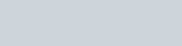0.01M MgC12Saturated Ba(OH)2 1.5M BaC12PH 7 and pH 10 buffer solutions LabPro unit with a pH probePipet250 ml beakers and Erlenmeyer flasks

Part 1: Determination of the Ksp for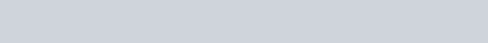and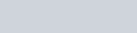# Experimental Procedure

l. Pour 20 ml of saturated solution into a 50 ml beaker.

1. Obtain and assemble a Vernier LabPro unit with a pH probe. You may use either a T183+ calculator or a laptop to control your LabPro unit.
2. Calibrate the pH probe using the pH 7 buffer and a pH 10 buffer as your two reference points. The calibration procedure is slightly different for groups that use a T183+ and groups that use a laptop computer to control the LabPro. See your instructor if you need assistance with the calibration.
3. Using the calibrated pH probe, record the pH of the saturated solution.
4. Rinse the pH probe with distilled water, use a Kimwipe to gently wipe off the drop of distilled water that remains on the tip of the probe, and repeat the pH measurement a second time.
5. Rinse the pH probe with distilled water, use a Kimwipe to gently wipe off the drop of distilled water that remains on the tip of the probe, and repeat the pH measurement a third time. Your final pH will be recorded as the average of your three pH measurements. Do not discard the Cu(OH)2 solution as you will need it for the next part of the experiment.
6. Repeat this procedure using saturated Ca(OH)2, Mg(OH)2, and Ba(OH)2 solutions.

Data Analysis (to be completed before moving on to the next section)

l . Using the average of the data from your three measurements of the pH of a saturated solution of calculate the concentration of hydroxide ion in the solution. Using the hydroxide ion concentration, use stoichiometry to determine the Ca2+ concentration.

Finally, using the Ca2+ and 01-1- concentrations, calculate the solubility product of

1. Using the average of the data from your three measurements of the pH of a saturated solution of calculate the concentration of hydroxide ion in the solution. Using the hydroxide ion concentration, use stoichiometry to determine the Cu concentration. Finally, using the Cu2+ and OH- concentrations, calculate the solubility product of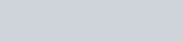1. Using the average of the data from your three measurements of the pH of a saturated solution ofBa(OH)2, calculate the concentration of hydroxide ion in the solution. Using the hydroxide ion concentration, use stoichiometry to determine the Ba 2+ concentration. Finally, using the Cu2+ and OH- concentrations, calculate the solubility product of1. Using the average of the data from your three measurements of the pH of a saturated solution of Mg(OH)2, calculate the concentration of hydroxide ion in the solution. Using the hydroxide ion concentration, use stoichiometry to determine the Mg concentration.

Finally, using the Mg2+ and OH- concentrations, calculate the solubility product of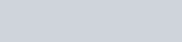1. Look up the published solubility product constants for each of the metal salts that you are using in this experiment. Calculate the percent error between the published value and your measured value. If your measured value is within 5% of the published value, use your value for the calculations in the second part of this experiment. Otherwise, use the published value for the remainder of your calculations.

## Part Il: Determination of the Effect of Common Ions on the pH of Hydroxide Solutions

Before you proceed with the data collection in this section, you need to calculate the pH of your saturated Ca(OH)2, Ba(OH) 2, and Mg(OH) 2 solution after the addition of a known amount of the corresponding metal chloride. Assume that the M2+ concentration from the chloride is the starting concentration in the equilibrium calculation for each hydroxide. You will be making the following additions:

l ) Add 20 ml of 0.5M caC12 to 20 ml of saturated Ca(OH) 2. 2) Add 20 ml of 1.5M BaC12 to 20 ml of saturated Ba(OH) 2.

3) Add 20 ml of 0.01M MgC12 to 20 ml of saturated Mg(OH) 2.

## Experimental Procedure

l . Add 20 ml of 0.5M CaC12 to your saturated solution from the first part of this experiment. Using your calibrated pH probe, measure the pH of the solution.

1. Add 20 ml of 0.01M MgC12 to your saturated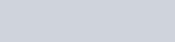solution from the first part of this experiment. Using your calibrated pH probe, measure the pH of the solution.
2. Add 20 ml of .5M BaC12 to your saturated Ba(OH)2 solution from the first part of this experiment. Using your calibrated pH probe, measure the pH of the solution.

## Data Analysis

l . Compare the calculated pH of your saturated Ca(OH)2 solution after the addition of CaC12 to the measured pH. Determine how well they compare by calculating the percent error between the two values.

1. Compare the calculated pH of your saturated Mg(OH)2 solution after the addition of MgC12 to the measured pH. Determine how well they compare by calculating the percent error between the two values.
2. Compare the calculated pH of your saturated solution after the addition of BaC12 to the measured pH. Determine how well they compare by calculating the percent error between the two values.
3. If metal nitrates were chosen for this part of the experiment instead of metal chlorides, how would this have affected your results?
4. You did not experiment with the common ion common effect using the compound Cu(OH)2. If you had added 20 ml of a solution of CuN03 to 20 ml of saturated Cu(OH)2, how will the pH of the solution be affected?